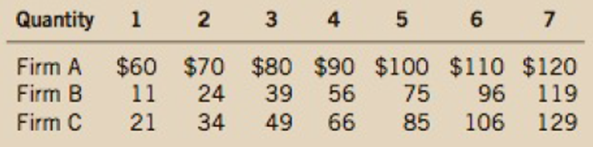Chapter 13, Problem 10PA

Chapter
Section
Textbook Problem

Consider the following table of long-run total costs for three different firms:Does each of these firms experience economies of scale or diseconomies of scale?To find additional study resources, visit cengagebrain.com,

Subpart (a):

To determine
Average total cost.

Explanation

Table -1 shows the data for total cost at different output levels:

Table -1

 Firm A Firm B Firm C Quantity TC ATC TC ATC TC ATC 1 60 11 21 2 70 12 34 3 80 13 49 4 90 14 66 5 100 15 85 6 110 16 106 7 120 17.14 129

Average total cost can be calculated by using the following formula:

Average total cost=(Total costQuantity) (4)

Substitute the respective value in the equation (1) to calculate the average total cost for firm A:

Subpart (b):

To determine
Firm are economies or diseconomies of scale.

Still sussing out bartleby?

Check out a sample textbook solution.

See a sample solution

The Solution to Your Study Problems

Bartleby provides explanations to thousands of textbook problems written by our experts, many with advanced degrees!

Get Started